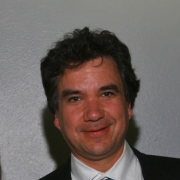# Doing statistics backwards: Structural Equation Modeling Using RAudience:
Topic:

Structural equation modeling (SEM) is a powerful tool for testing complex hypotheses. In SEM we start with a model (represented in a path diagram) and test it on the data; rather than the more common starting with the data and developing a model. SEM requires that we do statistics backwards. But SEM allows us to think about models in different ways, and test complex hypotheses that cannot (easily) be tested using other methods. In this talk I'll attempt to explain why you should be as excited about SEM as I can be.

I'll use an example of a problem that we had of developing a measured of neighborhood socio-economic status (NSES). NSES is measured using variables such as unemployment, median income, poverty rate, housing value, crowded housing. In a project looking at the effects of NSES, we needed a measure that was statistically justified and that exhibited stable measurement characteristics over time. Structural equation modeling presents a way to do this.

I'll also mention a song about structural equation modeling.

Room:
Los Angeles B
Time:
Sunday, February 23, 2014 - 16:30 to 17:30